# Exercise E7.1 Triangles NCERT Solutions Class 9

## Chapter 7 Ex.7.1 Question 1

In quadrilateral $$ACBD, AC = AD$$ and $$AB$$ bisects $$\angle A$$ (See the given figure). Show that \begin{align} \Delta ABC \cong \Delta ABD \end{align}. What can you say about $$BC$$ and $$BD$$?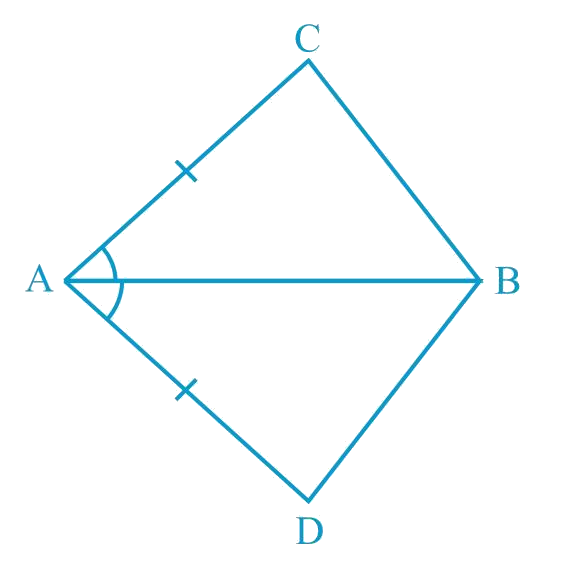### Solution

What is Known?

$$AC = AD$$ and $$AB$$ bisects

To prove:

$$\Delta {\text{ABC}} \cong \Delta {\text{ABD}}$$ and, what can be said about $$BC$$ and $$BD.$$

Reasoning:

We can show two sides and included angle of are equals to corresponding sides and included angle of by using SAS congruency criterion both triangles will be congruent and by CPCT, BC and BD will be equal.Steps:

In $$\Delta ABC$$ and $$\Delta ABD$$,

\begin{align} AC &= AD \text {(Given)}\\\\\Delta CAB &=\Delta DAB\\&(AB \text { bisects } \angle A)\\\\AB &= AB\\ &\text {(Common)}\\ \\ \therefore \Delta ABC &\cong \Delta ABD\\ &\text {(By congruence rule)}\\ \\\therefore BC&=BD\\&\left(\begin{array}{I} \text{By corresponding parts}\, \\ \text{of congruent triangles} \\ \end{array}\!\right)\end{align}

Therefore, $$BC$$ and $$BD$$ are of equal lengths.

## Chapter 7 Ex.7.1 Question 2

$$ABCD$$ is a quadrilateral in which $$AD = BC$$ and $$\angle DAB = \angle CBA$$ (See the given figure).

Prove that

(i) $$\Delta ABD \cong \Delta BAC$$

(ii) $$BD = AC$$

(iii) $$\Delta ABD=\Delta BAC$$

### Solution

What is Known?

${AD}={BC}\ \text{and}\ \angle {DAB}=\angle {CBA}$

To prove:

(i) $$\Delta {{ABD}} \cong \Delta {{BAC}}$$

(ii) $${{BD}} = {{AC}}$$

(iii) $$\angle {{ABD}} = \angle {{BAC}}$$

Reasoning:

We can show two sides and included of are equals to corresponding sides and included angle of by using $$\rm{SAS}$$ congruency criterion both triangles will be congruent. Then we can say corresponding parts of congruent triangle will be equal.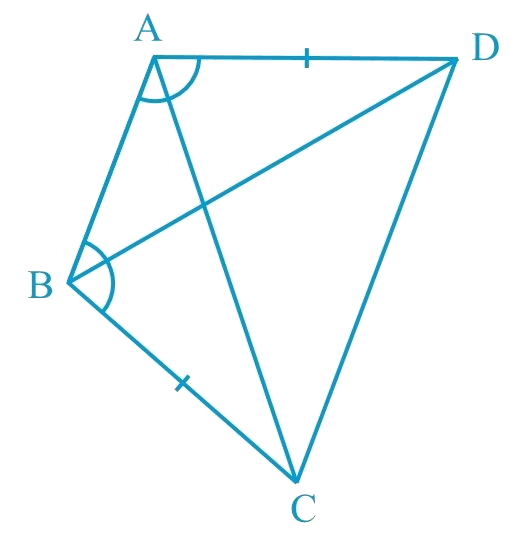Steps:

In $$\Delta ABD$$ and $$\Delta BAC$$,

\begin{align} AD &= BC \;\text{(Given)}\\\Delta DAB &=\Delta CBA \;\text{(Given)}\\AB &= BA \;\text{(Common)}\\\therefore \Delta ABD &\cong \Delta BAC\\& \text{(By congruence}\,\text{rule})\\\\\end{align}

\begin{align}\therefore BD &= AC \;\text{(By CPCT )}\\& \text{and}\\\angle ABD &=\angle BAC \;\text{(By CPCT )}\end{align}

## Chapter 7 Ex.7.1 Question 3

$$AD$$ and $$BC$$ are equal perpendiculars to a line segment $$AB$$ (See the given figure). Show that $$CD$$ bisects $$AB$$.

### Solution

What is Known?

$${\text{AD}} \bot {\text{AB, BC}} \bot {\text{AB}}\;{\text{and AD}} = {\text{BC}}$$

To prove:

$$CD$$ bisects $$AB$$ or $$OA = OB$$

Reasoning:

We can show two triangles $$OBC$$ and $$OAD$$ congruent by using AAS congruency rule and then we can say corresponding parts of congruent triangles will be equal.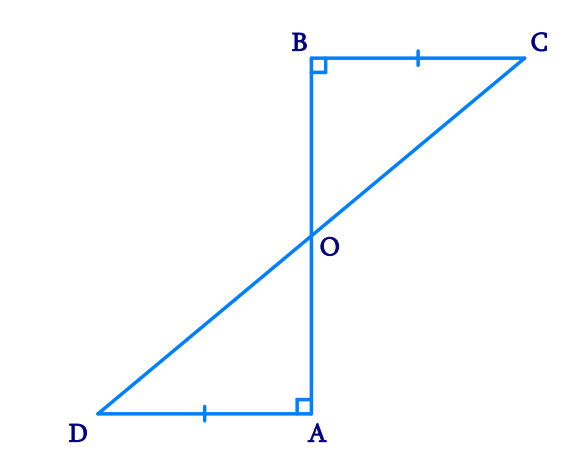Steps:

In $$\Delta BOC$$ and $$\Delta AOD$$,

\begin{align}\angle BOC& = \angle AOD\\\text{(Vertically opp}&\text{osite angles)}\\\\\angle CBO &= \angle DAO\text{(Each}\, 90^ {\circ})\\BC &= AD \text{(Given)}\\\therefore \Delta BOC &\cong \Delta AOD\\ \text{(AAS} \,& \text{congruence rule)}\\\\\therefore BO &= AO \text{(By CPCT)}\end{align}

Hence $$CD$$ bisects $$AB$$.

## Chapter 7 Ex.7.1 Question 4

$$l$$ and $$m$$ are two parallel lines intersected by another pair of parallel lines $$p$$ and $$q$$ (see the given figure). Show that $$\Delta ABC \cong \Delta CDA$$.

### Solution

What is Known?

$$l\parallel m\,\,\,and\,\,\,\,p\parallel q\,$$

To prove:

$$\Delta {\text{ABC}} \cong \Delta {\text{CDA}}{\text{.}}$$

Reasoning:

We can show both the triangles congruent by using ASA congruency criterion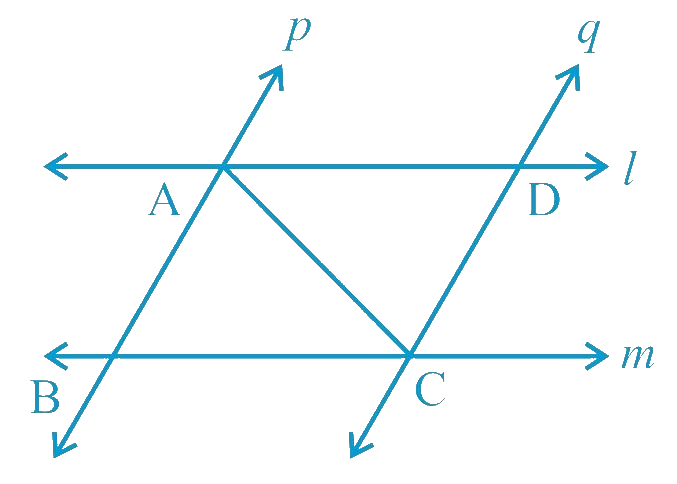Steps:

In $$\Delta ABC$$ and $$\Delta CDA$$,

\begin{align}&\angle BAC \,\text{and}\, \angle DCA \\&\text{(Alternate interior angles, as}(p \|q)\\\\AC &= CA \text{(Common)}\\&\angle BAC \text{and} \angle DCA \\&\text{(Alternate interior angles, as} (l \|m)\\\\&\therefore\,\, \Delta ABC \cong \Delta CDA \\&\text{(By ASA congruence rule)}\end{align}

## Chapter 7 Ex.7.1 Question 5

Line $$l$$ is the bisector of an angle $$\angle A$$ and $$\angle B$$ is any point on $$l$$. $$BP$$ and $$BQ$$ are perpendiculars from $$B$$ to the arms of $$\angle A$$ (see the given figure).

Show that:

(i) $$\Delta APB \cong \Delta AQB$$

(ii) $$BP = BQ$$ or $$B$$ is equidistant from the arms of $$\angle A$$.

### Solution

What is known?

$$l$$ is the bisector of an angle $$\angle {\text{A}}$$ and $$BP \bot AP\;{\text{and BQ}} \bot {\text{AQ}}$$

To prove:

$$\Delta {\text{APB}} \cong \Delta {\text{AQB}}$$ and $${\text{BP}}\,{\text{ = }}\,{\text{BQ}}$$ or B is equidistant from the arms of $$\angle {\text{A}}$$

Reasoning:

We can show two triangles APB and AQB congruent by using AAS congruency rule and then we can say corresponding parts of congruent triangles will be equal.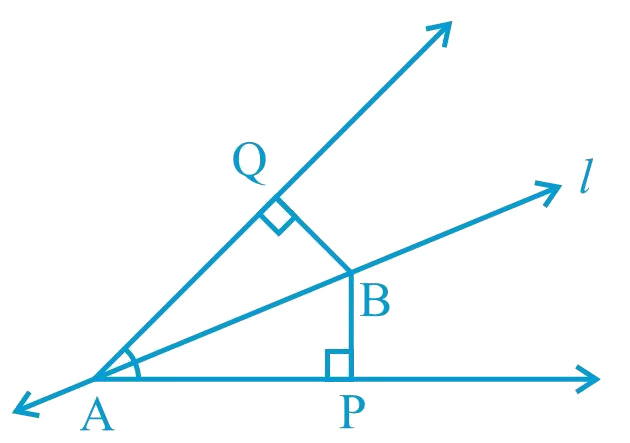Steps:

In $$\Delta APB$$ and $$\Delta AQB$$,

\begin{align}&\angle BAP= \angle BAQ \\&\text{(l) is the angle bisector of }(\angle A)\\\\&\angle APB = AQB ( \text{Each} \;90^ {\circ})\\AB&=AB \text{(Common)}\\&\therefore \Delta APB \cong \Delta AQB \\&\text{(By AAS congruence rule)}\\\\\therefore BP &= BQ (By CPCT)\end{align}

Or, it can be said that $$B$$ is equidistant from the arms of $$\angle A$$.

## Chapter 7 Ex.7.1 Question 6

In the given figure, $$AC = AE$$, $$AB = AD$$

and $$\angle BAD = \angle EAC$$.

Show that $$BC = DE$$.

### Solution

What is Known?

$$AC = AE, AB = AD$$ and

$$\angle BAD = \angle EAC.$$

To prove:

$$BC = DE.$$

Reasoning:

We can show two triangles BAC and DAE congruent by using SAS congruency rule and then we can say corresponding parts of congruent triangles will be equal. To show both triangles congruent two pair of equal sides are given and add angle DAC on both sides in given pair of angles BAD and angle EAC to find the included angle BAC and DAE.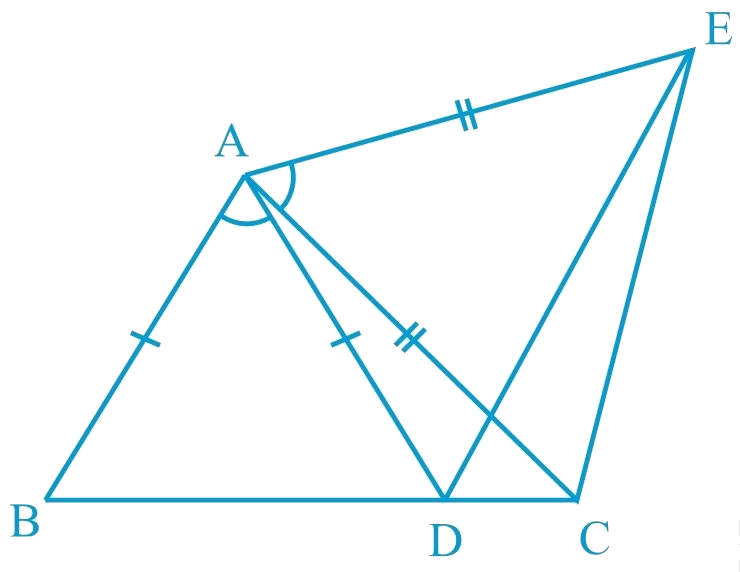Steps:

It is given that

$$\angle BAD = \angle EAC$$

$$\angle BAD\!+\!\angle DAC\!=\!\angle EAC\!+\!\angle DAC$$

$$\angle BAC = \angle DAE$$

In $$\Delta BAC$$ and $$\Delta DAE$$,

\begin{align} AB & =AD\,(\text{Given})\, \\ \angle BAC&=\angle DAE\, \\ & (\text{Proven}\,\,\text{above})\, \\ & \\ AC&=AE\,(\text{Given})\, \\ \therefore \Delta BAC&\cong \Delta DAE\, \\ (\text{By}\,\text{SAS cong}&\text{ruencerule}) \\ & \\\therefore BC & =DE\,(ByCPCT) \\ \end{align}

## Chapter 7 Ex.7.1 Question 7

$$AB$$ is a line segment and $$P$$ is its mid-point. $$D$$ and $$E$$ are points on the same side of $$AB$$

such that $$\angle BAD = \angle ABE$$ and

$$\angle EPA = \angle DPB$$ (See the given figure).

Show that:

(i) $$\Delta DAP \cong \Delta EBP$$

(ii) $$AD = BE$$

### Solution

What is Known?

$$P$$ is its mid-point of $$AB$$

$$\angle BAD = \angle ABE{\text{ }}and\;\angle EPA = \angle DPB$$,

To prove:

(i) $$\Delta DAP \cong \Delta EBP$$ and (ii) $$AD = BE$$ .

Reasoning:

We can show two triangles DAP and EBP congruent by using ASA congruency rule and then we can say corresponding parts of congruent triangles will be equal. To show both triangles congruent one pair of equal sides and one pair of equal angles are given and add angle EPD on both sides in given pair of angles EPA and angle DPB to find the other pair of angles APD and BPE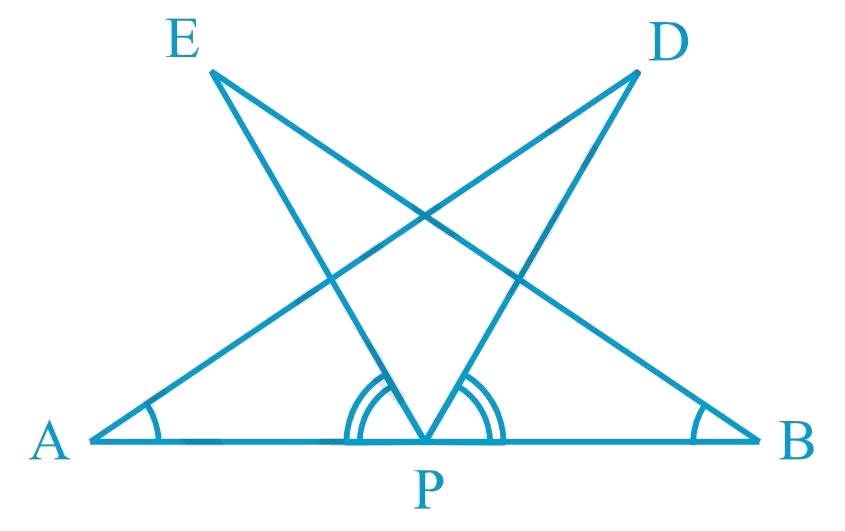Steps:

It is given that

$$\angle EPA = \angle DPB$$

$$\angle EPA + \angle DPE = \angle DPB + \angle DPE$$

$$\therefore \angle DPA = \angle EPB$$

In $$\Delta DAP$$ and $$\Delta EBP$$,

\begin{align}\angle DAP&=\angle EBP\,\,\,(\text{Given})\,\\AP&=BP\,\P\,\text{is}\,\text{mid}\,&\text{point}\,\text{of}\,\text{AB})\,\\\\\angle DPA&=\angle EPB\,\,\,(\text{From}\,\text{above})\,\,\\\therefore \Delta DAP&\cong \text{ }\!\!~\!\!\text{ }\Delta EBP\,\\&(\text{ASAcongruencerule})\,\\\\\therefore AD&=BE\,(By\,CPCT)\end{align} ## Chapter 7 Ex.7.1 Question 8 In right triangle \(ABC, right angled at $$C$$, $$M$$ is the mid-point of hypotenuse $$AB$$. $$C$$ is joined to $$M$$ and produced to a point $$D$$ such that $$DM = CM$$. Point $$D$$ is joined to point $$B$$ (see the given figure).

Show that:

(i) $$\Delta AMC \cong \Delta BMD$$

(ii) $$\angle DBC$$ is a right angle.

(iii) $$\Delta DBC \cong \Delta ACB$$

(iv) \begin{align}CM = \frac {1} {2}AB \end{align}

### Solution

What is Known?

$$M$$ is the mid-point of hypotenuse $$AB,$$

$$\angle C = 90^\circ$$ and $$DM = CM$$

To prove:

(i) $$\Delta AMC\cong \Delta BMD$$

(ii) $$\angle DBC$$ is a right angle.

(iii) $$\Delta DBC\cong \Delta ACB$$

(iv) $$CM=\frac{1}{2}AB$$

Reasoning:

We can show two triangles AMC and BMD congruent by using SAS congruency rule and then we can say corresponding parts of congruent triangles will be equal means angle ACM will equal to angle BDM, which are alternate interiors angle and can conclude DB is parallel to AC. Now it will help to find angle DBC by co-interior angles. Similarly triangles DBC and ACB will be congruent by using SAS criterion and CM will be half of AB by using M mid-point.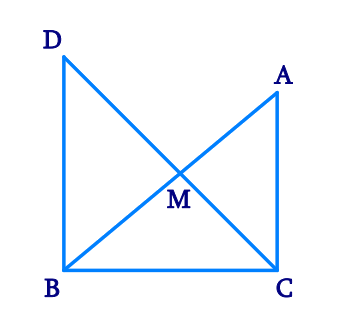Steps:

(i) In $$\Delta AMC$$ and $$\Delta BMD,$$

\begin{align}&AM = BM\\& (\text{M is the} \text{ mid-point of AB})\\\\&\angle AMC = \angle BMD\&\text{Vertically}\text{ opposite angles} )\\\\&\text{CM} = DM\left( \text{Given} \right)\\&\therefore \Delta AMC \cong \Delta BMD\\( &\text{By SAS}\text{ congruence rule})\\\\&\therefore AC = BD\left( \text{By CPCT} \right)\\&\text{And,}\,\angle ACM = \angle BDM\left( \text{By CPCT} \right)\end{align} (ii) \(\begin{align} \angle ACM\text{ }&=\angle BDM \end{align}

However, $$\angle ACM$$  and $$\angle BDM$$  are interior alternate angles.

Since alternate angles are equal,

It can be said that $$DB\, \|\,AC$$

\begin{align} \angle DBC+\angle ACB&={180}^{\circ}\\text{Co-interior}&\text{ angles }) \\\\ \angle DBC+{90}^{\circ}&={180}^{\circ} \\ \therefore \angle DBC&={90}^{\circ} \\ \end{align} (iii) \(\Delta DBC\,\text{ and}\,\Delta ACB

\begin{align}\\DB&=AC\;(\text{Already proved})\\\angle DBC&=\angle ACB\\text{Each }&\, \text{angle measures }90^{\circ})\\\\BC&=CB\;(\text{Common})\\\therefore \Delta DBC & \cong \Delta ACB\\(\text{SAS }&\text{congruence rule})\\\end{align} (iv) \( \Delta DBC\cong \Delta ACB

\begin{align}\\AB&=DC \;(\text{By }CPCT)\\AB&=2\,\text CM \\ \therefore CM&=\frac{1}{2}AB\\\end{align}

Instant doubt clearing with Cuemath Advanced Math Program